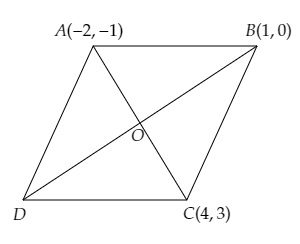# Three consecutive vertices of a parallelogram are $(-2, -1), (1, 0)$ and $(4, 3)$. Find the fourth vertex.

Given:

Three consecutive vertices of a parallelogram are $(-2, -1), (1, 0)$ and $(4, 3)$.

To do:

We have to find the fourth vertex.

Solution:

Let the coordinates of the three vertices are $A (-2, -1), B (1, 0)$ and $C (4, 3)$.
Let the fourth vertex be $D(x,y)$ and the diagonals $AC$ and $BD$ bisect each other at $O$.This implies,

$\mathrm{O}$ is the mid-point of $\mathrm{AC}$.

The coordinates of $\mathrm{O}$ are $(\frac{-2+4}{2}, \frac{-1+3}{2})$

$=(\frac{2}{2}, \frac{2}{2})$

$=(1,1)$

$\mathrm{O}$ is the mid-point of $\mathrm{BD}$.

The coordinates of $\mathrm{O}$ are $(\frac{1+x}{2}, \frac{0+y}{2})$

Therefore,

$(1,1)=(\frac{1+x}{2}, \frac{y}{2})$

On comparing, we get,

$\frac{1+x}{2}=1$

$1+x=1(2)$

$x=2-1=1$

Similarly,

$\frac{y}{2}=1$

$y=1(2)$

$y=2$

Therefore, the coordinates of the fourth vertex are $(1,2)$.

Updated on: 10-Oct-2022

25 Views